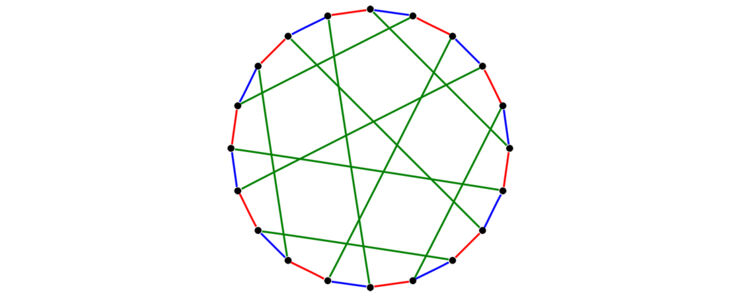# Graph Theory

The research group in graph theory at Linköping University is primarily interested in classic graph theory with a particular focus on graph coloring and Hamiltonian graph theory.A 3-edge-coloring of the Desargues graph. A mathematical graph (or network) is a natural model for a wide variety of phenomena and processes in the natural and social sciences, as well as in information and computer science, for studying pairwise relations between objects, including natural examples such as a road network or bonds in chemical molecules to more abstract phenomena such as links in social networks or data communication in a computer nework.

The idea of using graphs as mathematical models is usually attributed to the Swiss mathematician Euler and his well-known solution of the famous Königsberg bridge problem, but it was the equally famous Four color problem - Can every map be colored with four colors so that neighboring countries get different colors? - which was the driving force behind the fact that graph theory evolved into a mathematical discipline in its own right in the 20th century. In today's modern graph theory, there are connections to most other mathematical subjects; nevertheless, much of the research in graph theory remains strongly problem-oriented.

The research group in graph theory at Linköping University is primarily interested in classic graph theory with a particular focus on graph coloring and Hamiltonian graph theory.

In Hamiltonian graph theory we are primarily intersted in local conditions for Hamilton cycles or long cycles in graphs.

We study several graph coloring variants with a particular emphasis on edge colorings. A lot of the research is also devoted to questions on list coloring, where we investigate both deterministic and probabilistic models.

We collaborate with several other research groups and regularly welcome guests to the research group.